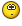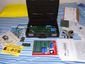# Here is a copy of Star Trek for 8k Apple I/Mimeo

9 posts / 0 new
Offline
Last seen: 2 weeks 8 hours ago
Joined: Oct 9 2011 - 12:54
Posts: 1352
Here is a copy of Star Trek for 8k Apple I/Mimeo

I had a very hard time finding a copy that would run on the 8k Mimeo/Apple I. The problem is all the versions available in AIFF or WAV format were "Enhanced" and needed more than the 4k available for basic programs. I did find the text of the version retyped by Pete Turnbull, but there were a lot of typos. Eventually between that copy and some other sources I managed to create a version that worked correctly. If anyone finds any problems or errors let me know so I can fix them.

Here is the code, instructions and a file for your iPod or cassette player to use with the ACI...

And the code...

LOMEM = 768

10 DIM C\$(6),D\$(15)
20 Q=640:S=704:D\$=" * >!<+++<*>"
30 INPUT "TYPE A NUMBER ",R
40 FOR J=1 TO R:C= RND (256): NEXT J
50 B=Q: GOSUB 1100
60 S1=75:N=S1:V=1:L=10: GOSUB 1200
70 B1=2:N=B1:V=10:L=20: GOSUB 1200
80 K1=7:N=K1:V=20:L=40: GOSUB 1200
90 T=15: GOSUB 1300:E0= RND (4096): GOSUB 1400: GOSUB 1000
110 PRINT : INPUT "COMMAND ",C: IF C<0 OR C>5 THEN 110: GOTO 100*C+200
200 INPUT "VECTOR ",X,Y:X0=X0+X:Y0=Y0+Y:E1=E1- ABS (X)- ABS(Y)
210 IF X0<0 OR X0>63 OR Y0<0 OR Y0>63 THEN 250
220 E0=E0+X+64*Y:Q1=Q0:S1=S0: GOSUB 1400: IF Q0=Q1 THEN 255
230 E1=E1-25:T=T-1: GOSUB 1000: GOSUB 1400: IF T>=0 THEN 260
240 C\$="TIME": GOTO 980
250 C\$="GALAXY": GOTO 980
255 POKE S+S1,0: GOSUB 1050
260 GOSUB 1800: IF B2=0 THEN 290: IF ABS (X6-X2)+ ABS (Y6-Y2)#1 THEN 290
270 PRINT "- DOCKED -": GOSUB 1300:B1=B1-1:B2=0
280 POKE Q+Q0, PEEK (Q+Q0)-10: POKE S+X6+8*Y6,0: GOTO 110
290 IF K2#0 THEN GOSUB 550: GOTO 110
300 C\$="SHORT": GOSUB 350: GOSUB 2000: GOTO 110
350 PRINT C\$;" RANGE SENSOR SCAN": GOSUB 360: RETURN
360 PRINT "FOR QUADRANT ";X1;",";Y1: RETURN
400 C\$="LONG": GOSUB 350:N=3: GOSUB 880
410 FOR Y=Y1+1 TO Y1-1 STEP -1:F2=0: IF Y<0 OR Y>7 THEN F2=1: GOSUB 470: GOSUB 480
420 FOR X=X1-1 TO X1+1:F1=0: IF X<0 OR X>7 THEN F1=1
430 IF F1=0 AND F2=0 THEN 440:PRINT C\$;: GOTO 460
440 Q9=X+8*Y: GOSUB 1500: POKE Q+Q9,C9+40: PRINT "! ";K2;B2;S2;" ";
460 NEXTPRINT "!": GOSUB 480: NEXT Y: GOSUB 470: GOSUB 890: GOTO 110
470 C\$="+-----": GOSUB 1600: RETURN
480 C\$="! ": GOSUB 1600: RETURN
500 IF K2=0 THEN 690: GOSUB 790: PRINT "ENERGY: ";E1: INPUT "FIRE ",C: IF C<1 THEN 110
520 E1=E1-C: GOSUB 1800:K5=K5-C-(R*9): IF K5>0 THEN 530: GOSUB 1900: GOTO 110
530 GOSUB 550: GOTO 500
550 J5=K5/5:K5=K5-J5:E1=E1-J5/R: PRINT J5/R;" UNITS OF PHASER DAMAGE": GOSUB 1800: RETURN
600 IF K2=0 THEN 690: IF E2#0 THEN 610: PRINT "NO MORE TORPEDOES" : GOTO 110
610 E2=E2-1: IF R> RND (15) THEN 640: GOSUB 1900: GOTO 110
640 PRINT "YOU MISSED!": GOSUB 550: GOTO 110
690 PRINT "NOTHING TO SHOOT AT!": GOTO 110
700 INPUT "COMPUTER REQUEST ",C: IF C=0 THEN 800
710 PRINT "STATUS REPORT:": PRINT
720 GOSUB 790: PRINT "KLINGONS =",K1
730 GOSUB 790: PRINT "STARDATES =",T
740 GOSUB 790: PRINT "STARBASES =",B1
750 GOSUB 790: PRINT "TORPEDOES =",E2
760 GOSUB 790: PRINT "ENERGY =",E1
770 GOTO 110
790 PRINT "REMAINING ";: RETURN
800 PRINT " GALACTIC MAP": PRINT " ";: GOSUB 360:C\$=" ---": N=8
810 GOSUB 880: FOR Y=7 TO 0 STEP -1: GOSUB 1600
820 FOR X=0 TO 7:Q9=X+8*Y: GOSUB 1500:P= PEEK (Q+Q9)
830 IF P>39 THEN 840: PRINT " ";: GOTO 850
840 PRINT " ";K2;B2;S2;
850 NEXTPRINT
860 NEXT Y: GOSUB 1600: GOSUB 890: GOTO 110
880 B8=B2:K8=K2: RETURN
890 B2=B8:K2=K8: RETURN
980 PRINT : PRINT "-- OUT OF ";C\$;" --"
990 PRINT "YOU LEFT ";K1;" KLINGONS": END
1000 B=S: GOSUB 1100:Q9=Q0: GOSUB 1500: POKE Q+Q9,C9+40
1010 N=S2:V=1:L=2: GOSUB 1200
1020 N=K2:V=3:L=4: GOSUB 1200:K5=100:Y5=I/8:X5=I-8*Y5
1030 N=B2:V=2:L=3: GOSUB 1200:Y6=I/8:X6=I-8*Y6
1035 C\$="GREEN": IF K2#0 THEN C\$="RED!": PRINT : PRINT "STARDATE: ";3278-T;", CONDITION: ";C\$
1040 PRINT "QUADRANT ";X1;",";Y1;" - SECTOR ";X2;",";Y2
1050 P= PEEK (S+S0): POKE S+S0,4: IF P=0 THEN RETURN
1060 PRINT : PRINT "*** COLLISION WITH A ";
1070 IF P=1 THEN PRINT "STAR";: IF P=2 THEN PRINT "STARBASE";: IF P=3 THEN PRINT "KLINGON";
1080 PRINT " ***": IF P=3 THEN K1=K1-1: GOTO 990
1100 FOR K=0 TO 63: POKE B+K,0: NEXT K: RETURN
1200 IF N=0 THEN RETURN : FOR K=1 TO N
1210 I= RND (64):P= PEEK (B+I): IF P+V>=L THEN 1210: POKE B+I,P+V: NEXT K: RETURN
1300 E1=500:E2=3: RETURN
1400 Y0=E0/64:X0=E0-64*Y0:Y1=Y0/8:X1=X0/8:Y2=Y0-8*Y1:X2=X0-8*X1
1410 Q0=X1+8*Y1:S0=X2+8*Y2:R= ABS(X5-X2)+ ABS (Y5-Y2): RETURN
1500 C9= PEEK (Q+Q9): IF C9>=40 THEN C9=C9-40
1510 S2=C9-10*(C9/10):K2=C9/20: B2=(C9-S2-20*K2)/10: RETURN
1600 FOR J=1 TO N: PRINT C\$;: NEXT J: PRINT C\$(1,1): RETURN
1800 IF E1>0 THEN RETURN
1810 C\$="ENERGY": GOTO 980
1900 PRINT "*** BOOM ***":K1=K1-1:K2=0: POKE Q+Q0, PEEK (Q+Q0)-20: POKE S+X5+8*Y5,0
1910 IF K1 THEN RETURN : PRINT: PRINT "MISSION ACCOMPLISHED!" : END
2000 C\$="+---":N=8: GOSUB 1600
2010 FOR Y=7 TO 0 STEP -1: FOR X=0 TO 7
2020 P=3* PEEK (S+X+8*Y)+1: IF X=0 THEN PRINT "!";: IF X#0 THEN PRINT " ";: PRINT D\$(P,P+2);
2030 NEXTPRINT "!": IF Y=0 THEN 2040: PRINT "+";: TAB 33: PRINT "+"
2040 NEXT Y: GOSUB 1600: RETURN

Offline
Last seen: 2 weeks 8 hours ago
Joined: Oct 9 2011 - 12:54
Posts: 1352
Forgot to post this :)Offline
Last seen: 5 months 1 week ago
Joined: Nov 21 2010 - 15:30
Posts: 248
Re: Here is a copy of Star Trek for 8k Apple I/Mimeo

Hi Corey,
Nice work!
To load the star trek program you have to before load the basic? So this is a 4k version WOW.

Offline
Last seen: 2 weeks 8 hours ago
Joined: Oct 9 2011 - 12:54
Posts: 1352
Re: Here is a copy of Star Trek for 8k Apple I/Mimeo

The credit should go to Pete Turnbull who retyped this thing from an old magazine. He did have a lot of typos. Also credit should go to Open Emulator. I actually worked it out on OpenEmulator and even created the wave file for the ACI using OpenEmulator. Then I went over to the Mimeo to test it (Play for a while). Until I get my Mimeo in a case I don't want to turn it on a lot just to be safe since the keyboard is sitting on the encoder and I don't want it to break anything by typing too hard.

And yes it is very efficient code with a lot of re-use to fit in the 4k left after you load basic. Actually less than 4k as LOMEM is set to 768 and HIMEM is default of 4096.

Cheers,
CoreyOffline
Last seen: 5 months 1 week ago
Joined: Nov 21 2010 - 15:30
Posts: 248
Re: Here is a copy of Star Trek for 8k Apple I/Mimeo

This is great!
I think i will put my mimeo 1 inside a brefcase like the old style : ).Offline
Last seen: 5 months 1 week ago
Joined: Nov 21 2010 - 15:30
Posts: 248
Re: Here is a copy of Star Trek for 8k Apple I/Mimeo

Corey,
I have tried your startek it works, but when i do command 1 i get a string error after the drawing.

Offline
Last seen: 2 weeks 8 hours ago
Joined: Oct 9 2011 - 12:54
Posts: 1352
Re: Here is a copy of Star Trek for 8k Apple I/Mimeo

Did you load this from Audio file? Type it in? or load it through Serial I/O using using a serial keyboard adapter?

If you typed it in I would double check for typos. If you loaded from audio file, did you use an iPod or direct from a PC? Check your volume you may have an error in the load.
If you used the Serial I/O maybe there is a control character or an error in the load. Try play with the serial settings and clean up the "Text" by pasting it into a simple text editor first.

Let me know...

Cheers,
Corey

Offline
Last seen: 2 weeks 8 hours ago
Joined: Oct 9 2011 - 12:54
Posts: 1352
Re: Here is a copy of Star Trek for 8k Apple I/Mimeo

Well apparently you can't cut and paste the code from the website page and upload it over a serial connection it does indeed produce that error due to some control character being carried over. It's not worth figuring out, instead this is a link to a clean text file version....

Apple 8k Trek Link to Basic Code

I wanted to add some credit here to OpenEmulator... I used it to figure out it was not a good thing to cut and paste from the text in this thread to load it....Offline
Last seen: 5 months 1 week ago
Joined: Nov 21 2010 - 15:30
Posts: 248
Re: Here is a copy of Star Trek for 8k Apple I/Mimeo

Thank you this version works!!